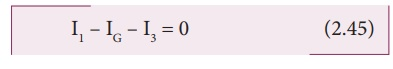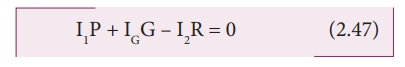Home | | Physics 12th Std | Wheatstone‚Äôs bridge

# Wheatstone‚Äôs bridge

An important application of Kirchhoff‚Äôs rules is the Wheatstone‚Äôs bridge.

Wheatstone‚Äôs bridge

An important application of Kirchhoff‚Äôs rules is the Wheatstone‚Äôs bridge. It is used to compare resistances and also helps in determining the unknown resistance in electrical network. The bridge consists of four resistances P, Q, R and S connected as shown in Figure 2.25. A galvanometer G is connected between the points B and D. The battery is connected between the points A and C. The current through the galvanometer is IG and its resistance is G.

Applying Kirchhoff‚Äôs current rule to junction BApplying  Kirchhoff‚Äôs  current  rule  to junction D,Applying Kirchhoff‚Äôs voltage rule to loop ABDA,Applying Kirchhoff‚Äôs voltage rule to loop ABCDA,When the points B and D are at the same potential, the bridge is said to be balanced. As there is no potential difference between B and D, no current flows through galvanometer (IG = 0). Substituting IG = 0 in equation (2.45), (2.46) and (2.47), we getSubstituting  the  equation  (2.49)  and (2.50) in equation (2.48)Dividing equation (2.52) by equation (2.51), we getThis is the bridge balance condition. Only under this condition, galvanometer shows null deflection. Suppose we know the values of two adjacent resistances, the other two resistances can be compared. If three of the resistances are known, the value of unknown resistance (fourth one) can be determined.

EXAMPLE 2.23

In a Wheatstone‚Äôs bridge P = 100 Œ©, Q = 1000 Œ© and R = 40 Œ©. If the galvanometer shows zero deflection, determine the value of S.

SolutionEXAMPLE 2.24

What is the value of x when the Wheatstone‚Äôs network is balanced?

P = 500 Œ©, Q = 800 Œ©, R = x + 400, S = 1000 Œ©Solution

P/Q = R/Sx + 400 = 0.625 √ó 1000

x + 400 = 625

x = 625 ‚Äì 400